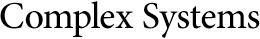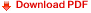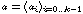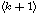Dynamical Behavior of a Neural Automaton with MemoryMichel Cosnard
Driss Moumida
INPG, Laboratoire TIM3, 46 avenue Felix Viallet,
38031 Grenoble Cedex, France

Eric Goles
Department of Mathematics, Engineering School, University of Chile,
Casilla 170/3, Correo 3, Santiago, Chile

Thierry de St. Pierre
Laboratoire de Robotique, CRIIF, Paris VI,
4 Place Jussieu, 75005, France

Abstract

We study the dynamics of an automaton with memory whose equation is the following:wheredenotes the coupling coefficients vector. We show that ifis symmetric, then we can introduce an energy operator; thereby we state the periods of the automaton always divideand give a bound of the transient. We also study the case of reversible systems and characterize reversibility versus the coupling coefficients. Thereafter, we give some results about the pivot sums systems. Some conjectures concerning the general case are given.